# NGS for NGS

##### Posted on Jan 11, 2020 (Update: Jan 15, 2020)

This post is based on the talk, Next-Generation Statistical Methods for Association Analysis of Now-Generation Sequencing Studies, given by Dr. Xiang Zhan at the Department of Statistics and Data Science, Southern University of Science and Technology on Jan. 05, 2020.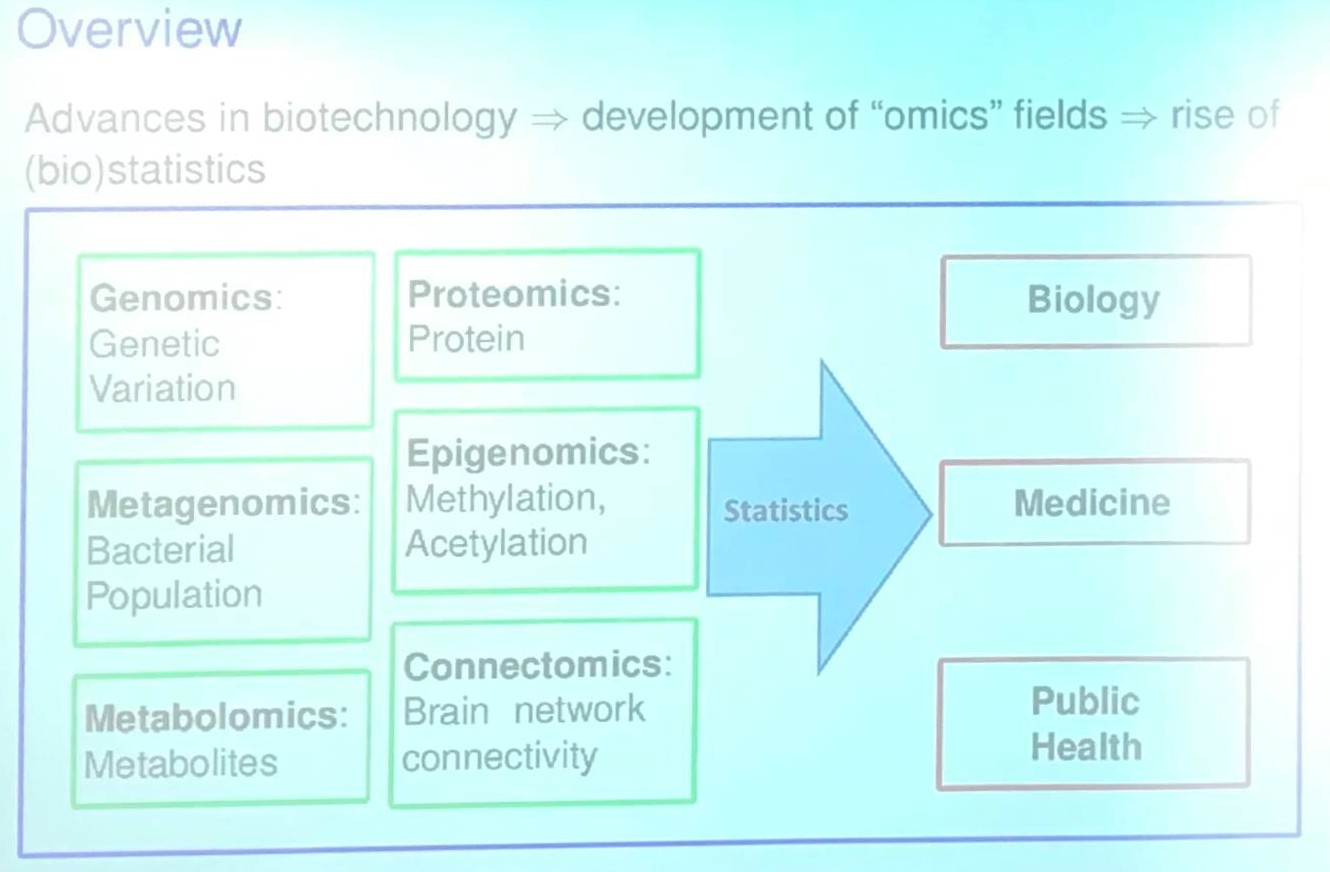## Project I: Statistical Methods for Set-based Omics Association Analysis

Goal: Test

$H_0: \beta_1 = \cdots = \beta_p = 0$

in

$y=X\alpha + Z_1\beta_1 + \cdots + Z_p\beta_p + \epsilon\,.$

### KAT (Kernel-based Association Test)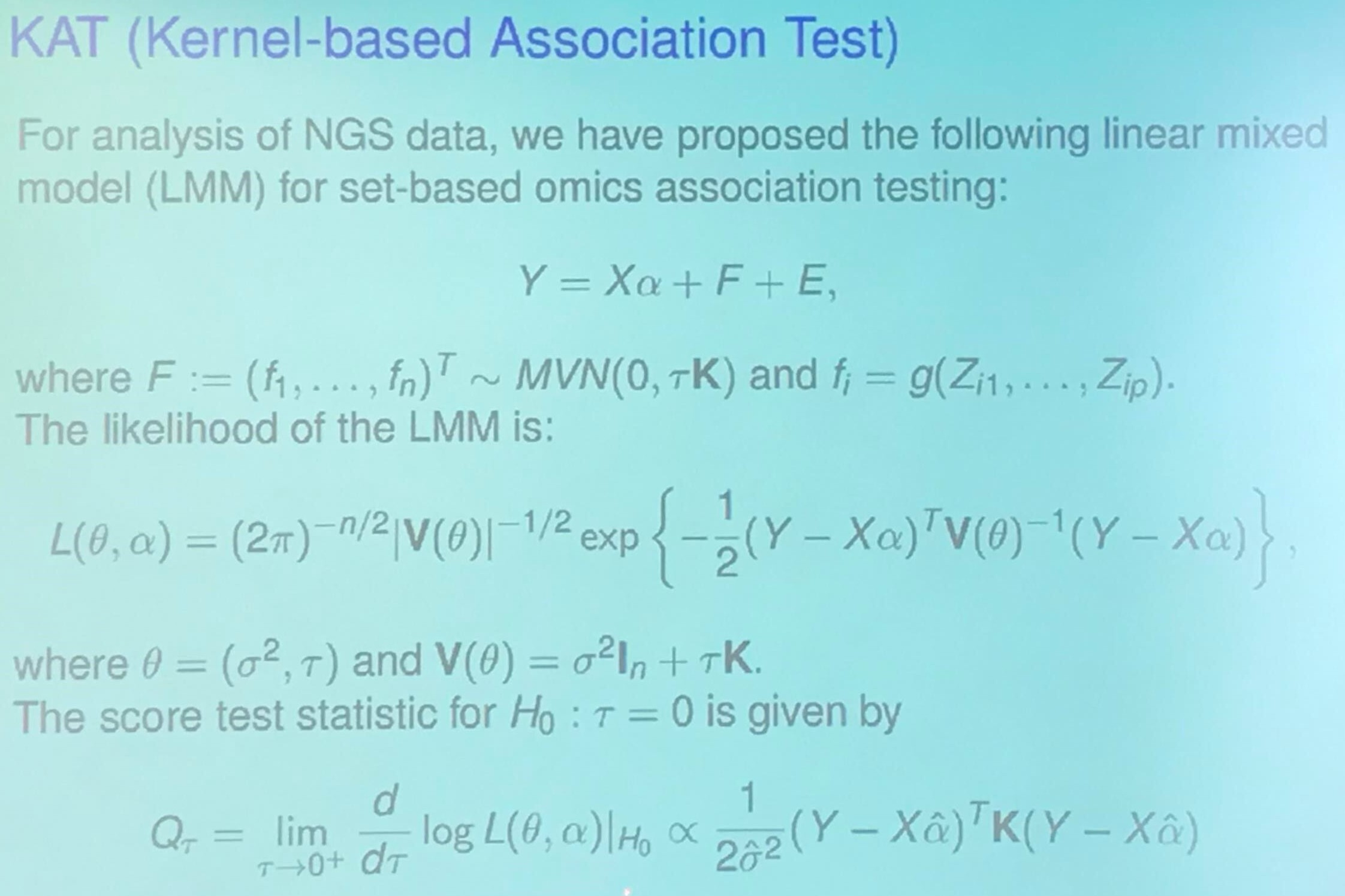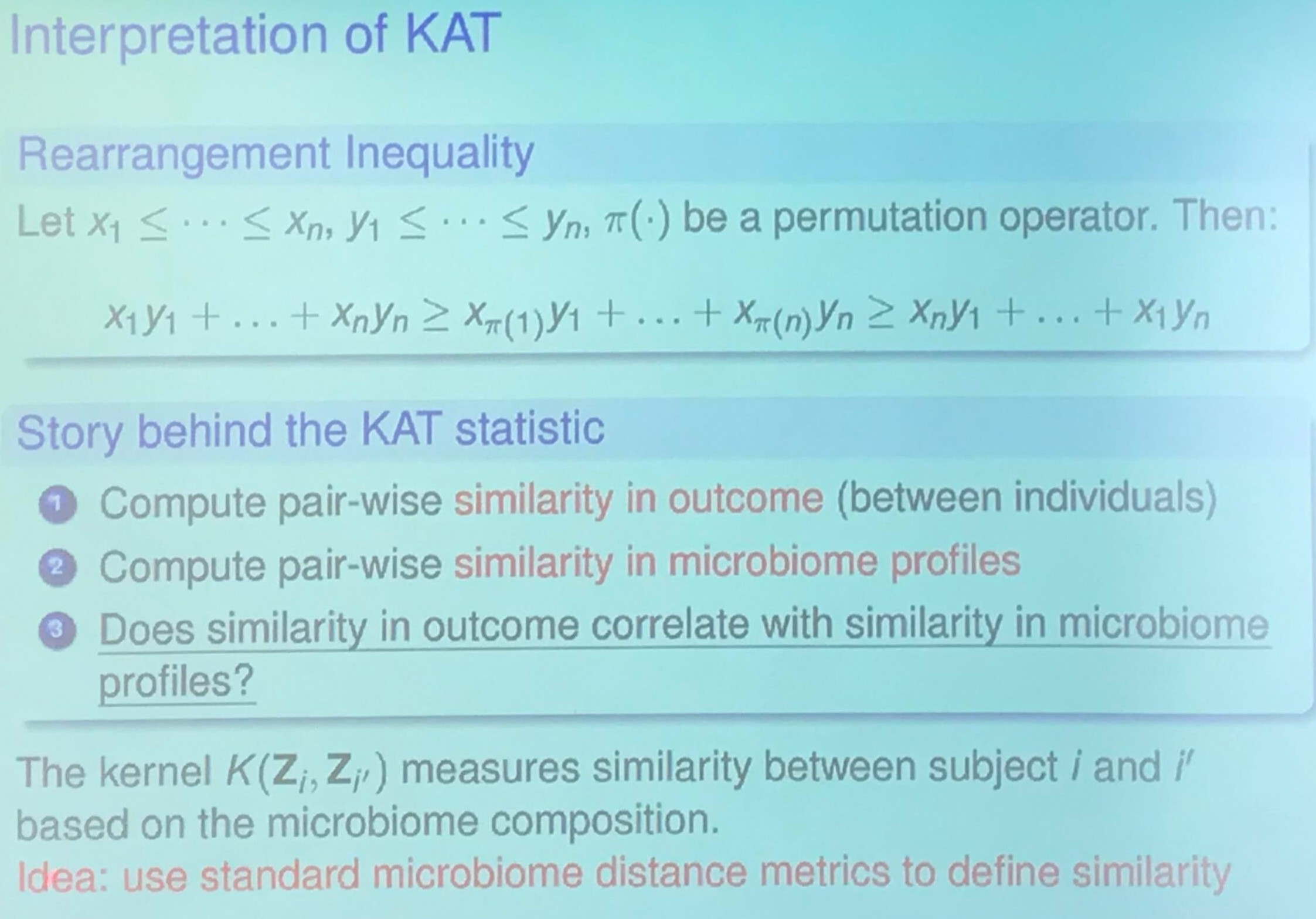I am confused about the relationship between Rearrangement Inequality and KAT statistic. My guess is that it wants to show higher score implies higher correlation.

### Microbiome Data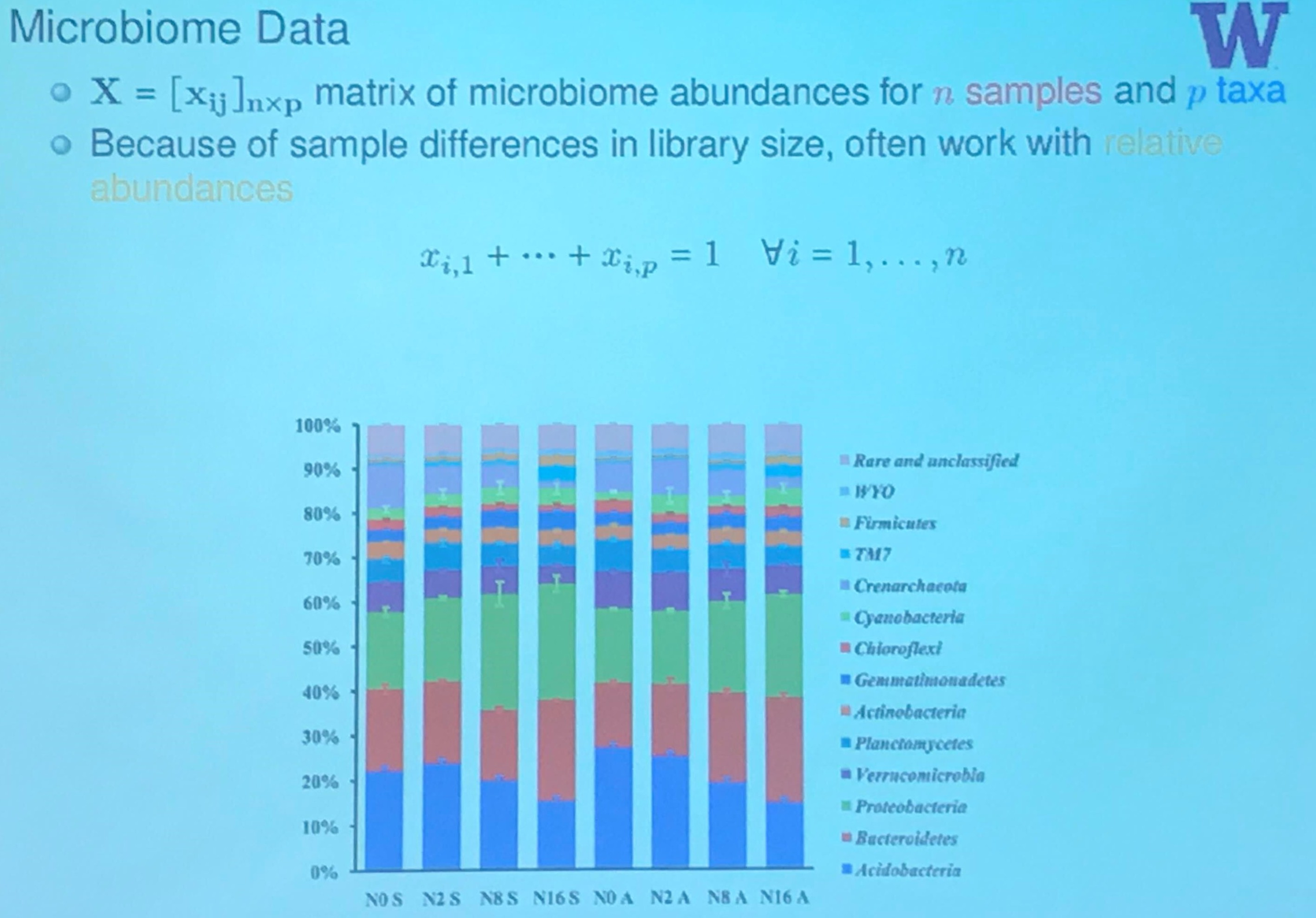(Actually, this slide is from another speak in that conference, I will have a post later.)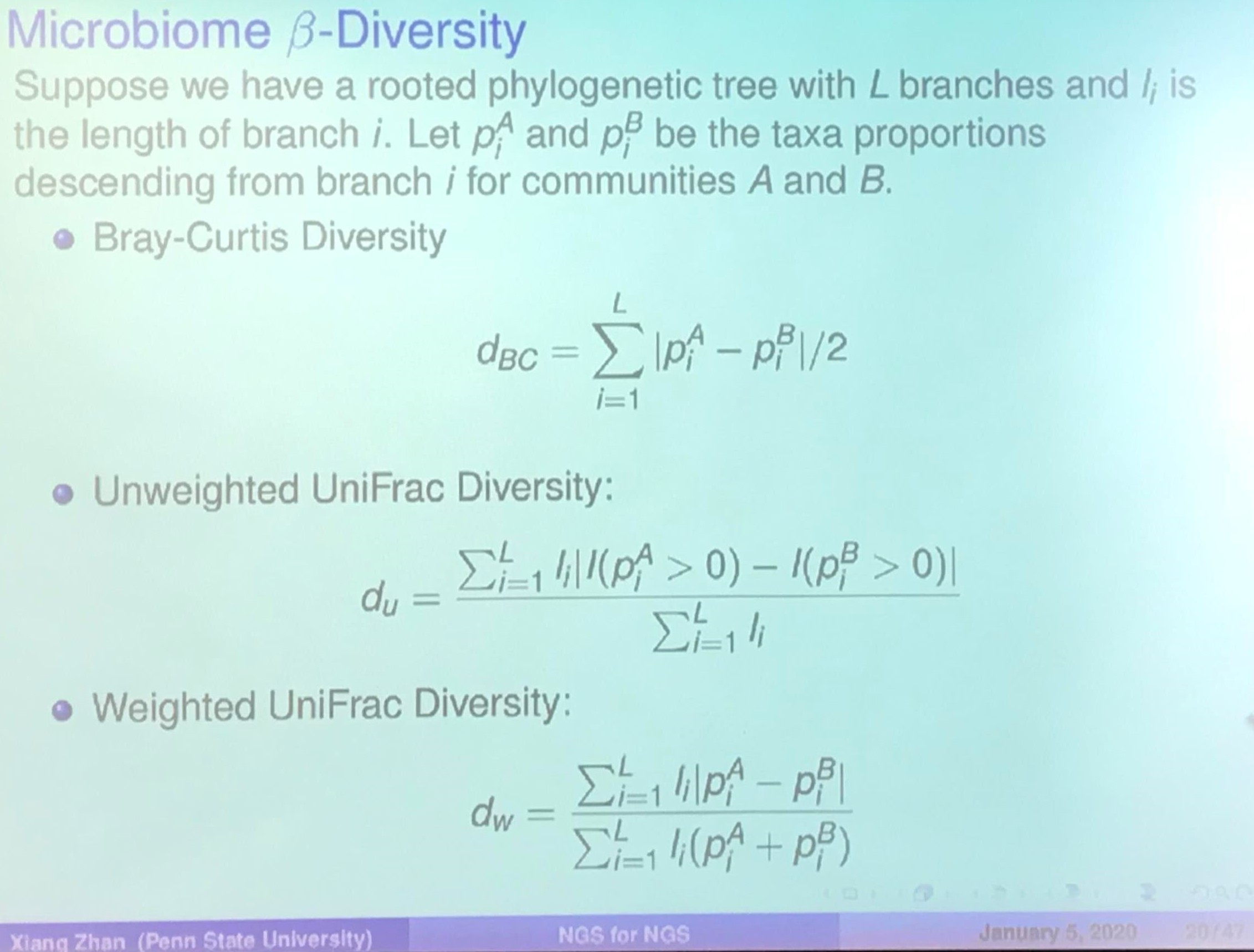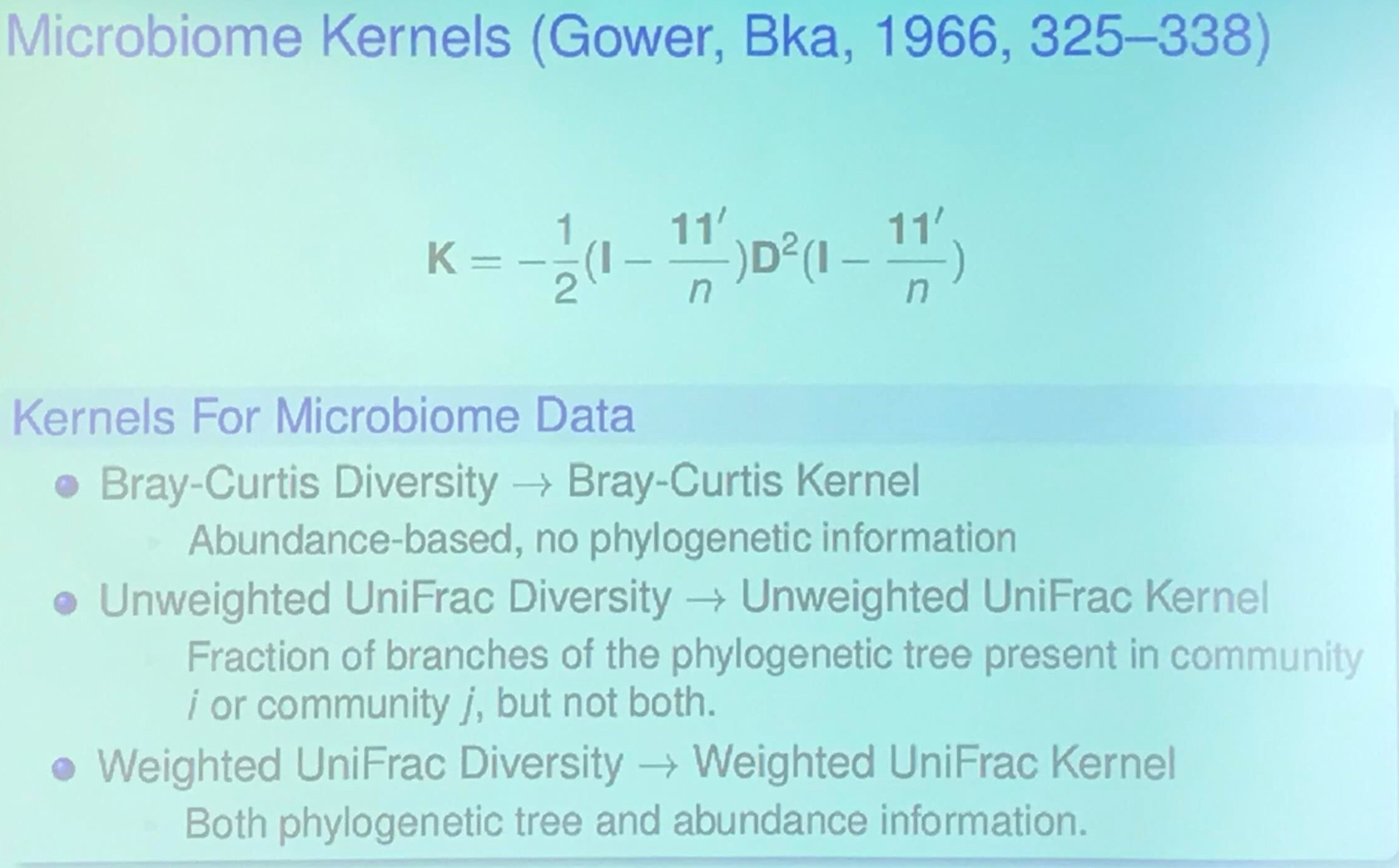An OTU table is a matrix that gives the number of reads per sample per OTU. One entry in the table is usually a number of reads, also called a “count”, or a frequency in the range 0.0 to 1.0.### Host Transcriptome and Microbiome Study (TODO)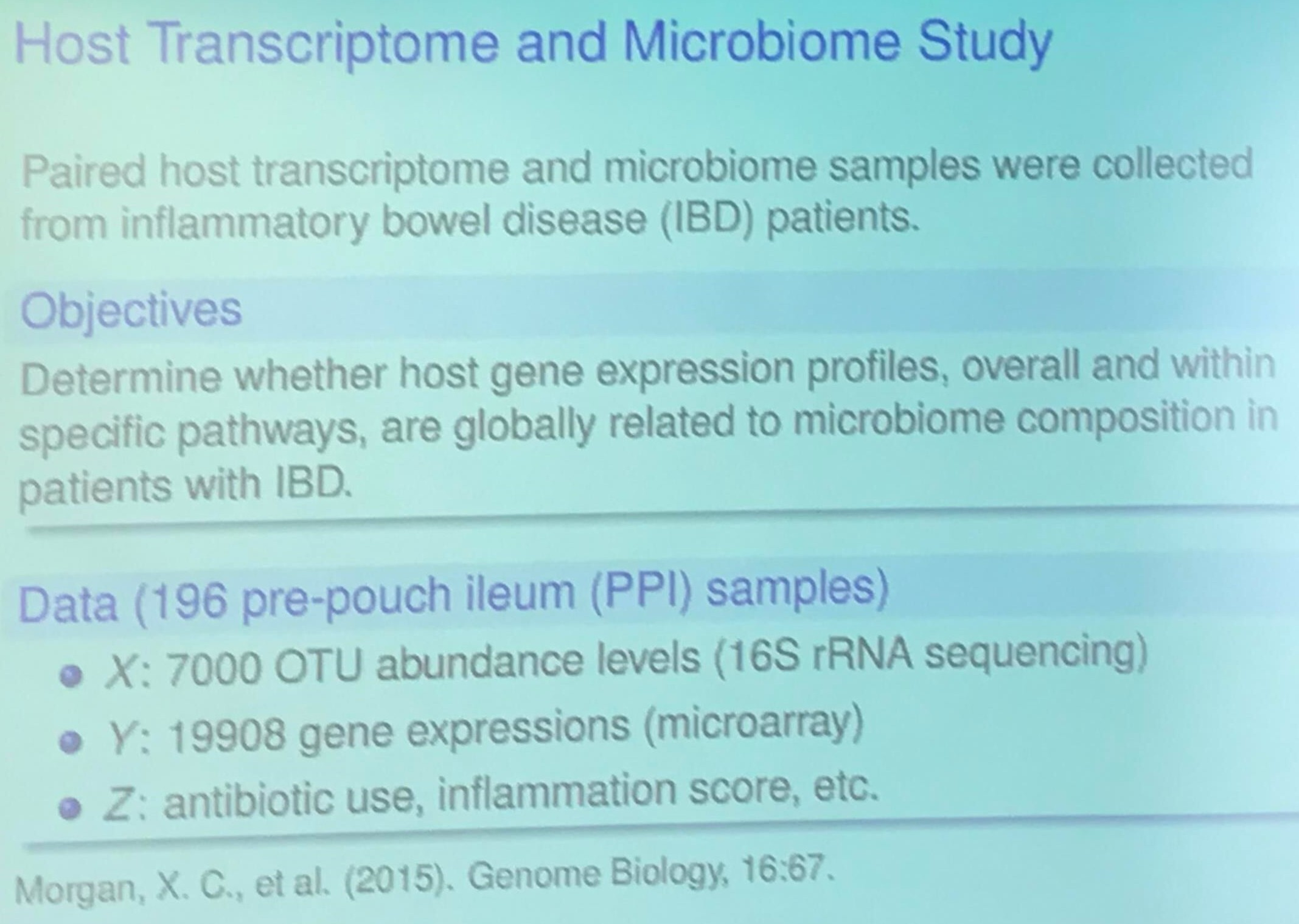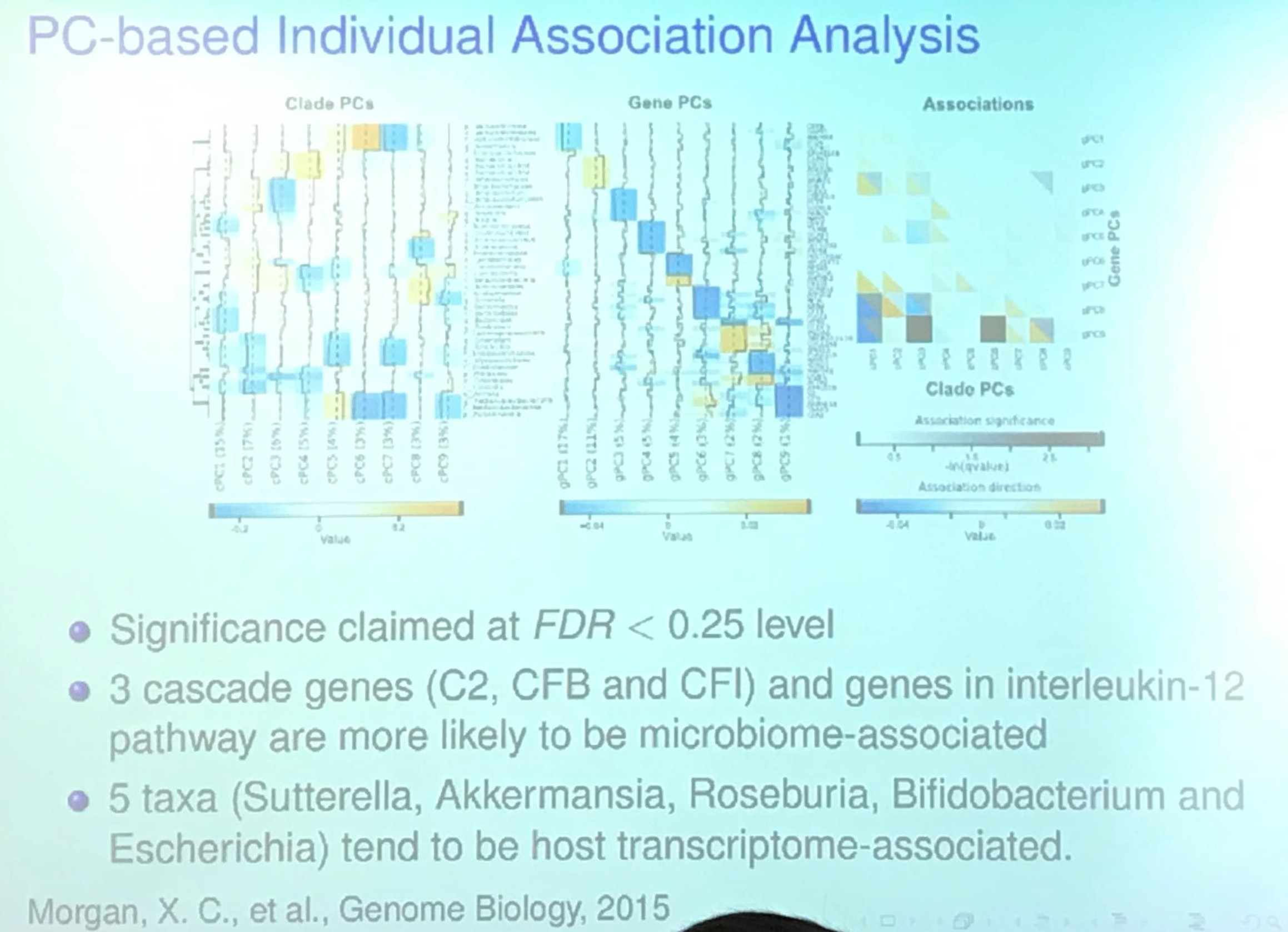## Project II: Variable Selection Methods for Prioritizing Omics Association Signals

Goal: Identify $\{j:\beta_j\neq 0, j=1,\ldots, p\}$ in

$y = \beta_1X_1 + \cdots +\beta_pX_p + \epsilon\,.$

### Identifying Taxa via Multiple Testing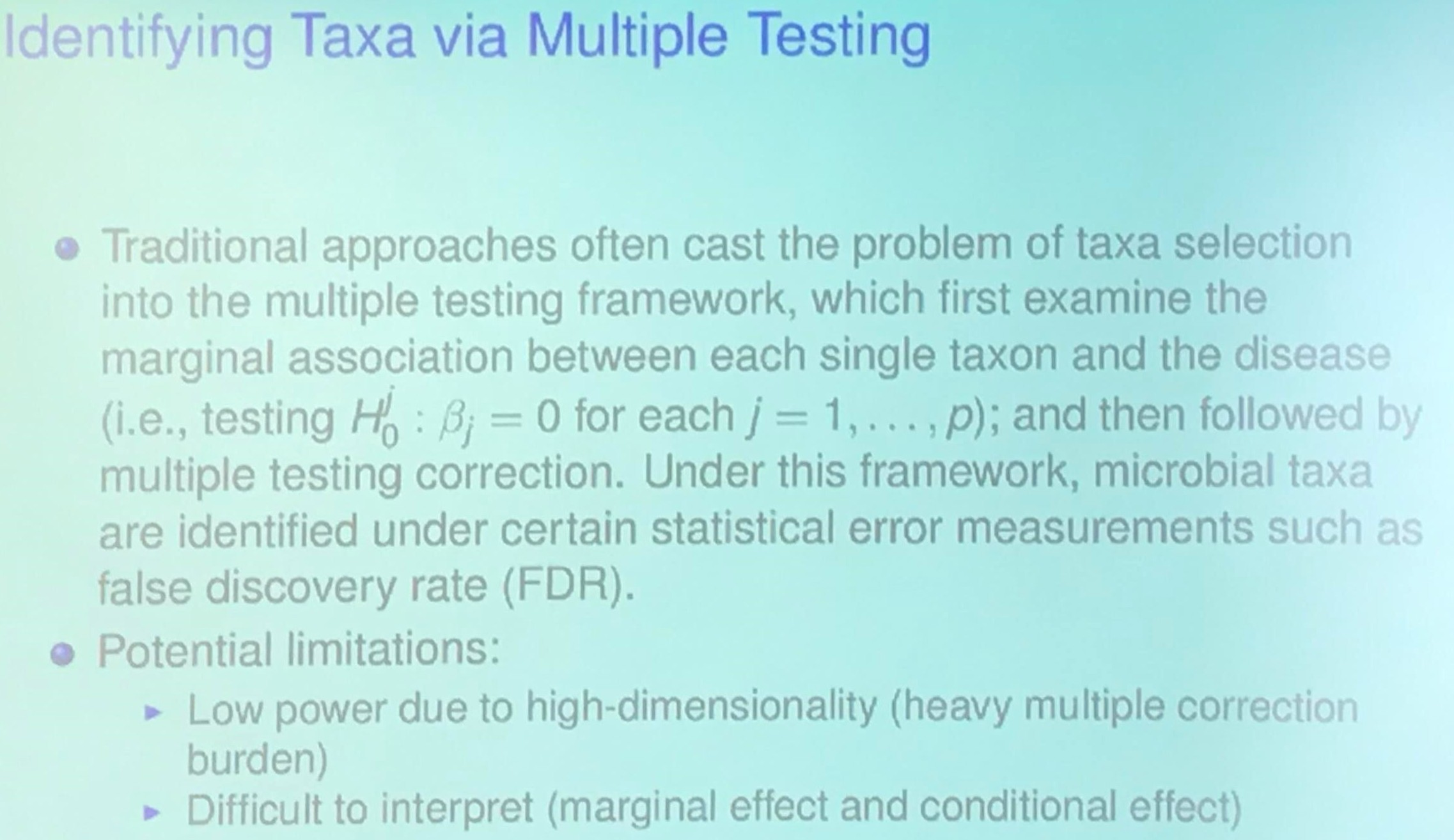Wang, Q., Liu, X., Jiang, L., Cao, Y., Zhan, X., Griffin, C. H., & Wu, R. (2019). Interrogation of Internal Workings in Microbial Community Assembly: Play a Game through a Behavioral Network? MSystems, 4(5).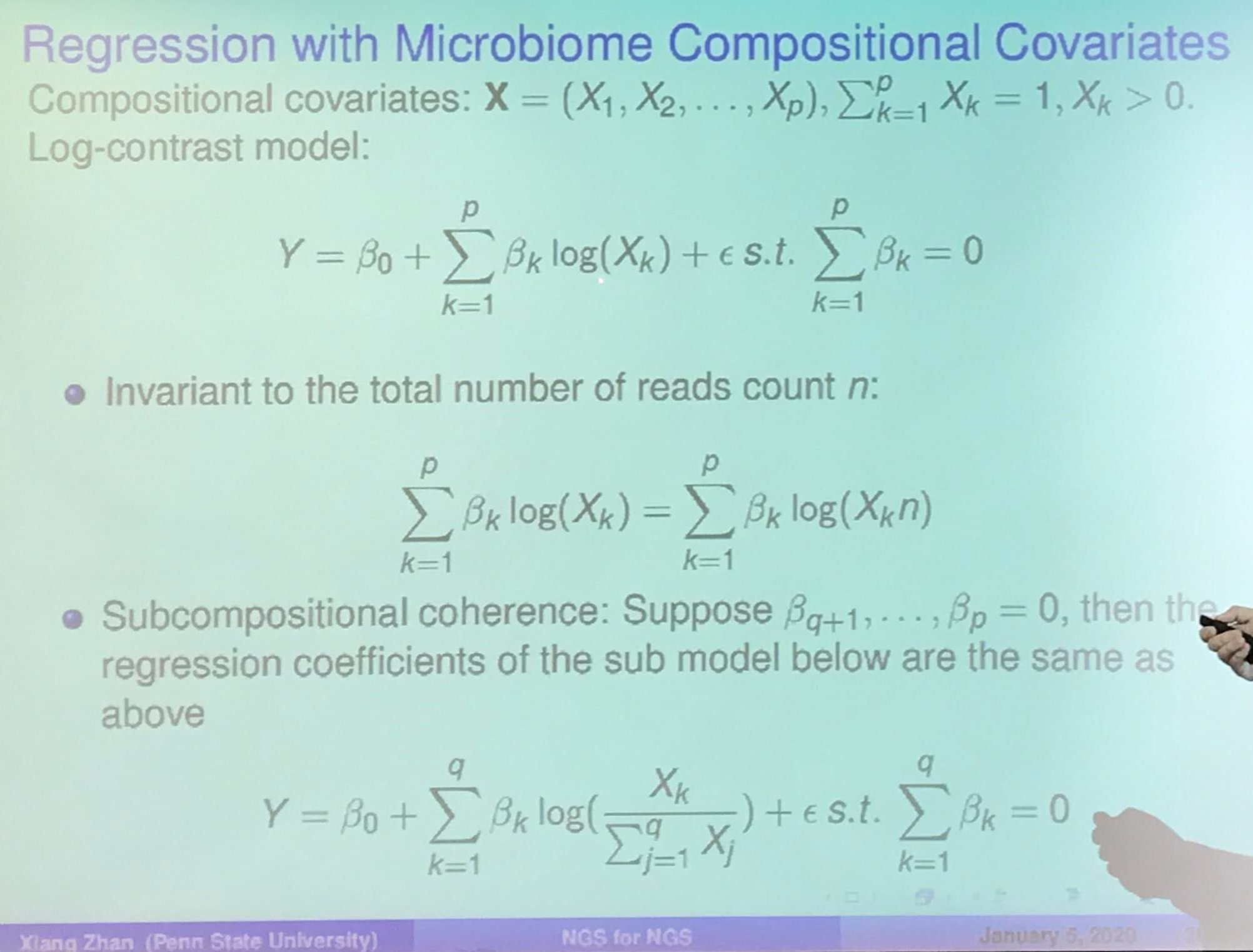### Knockoff Filter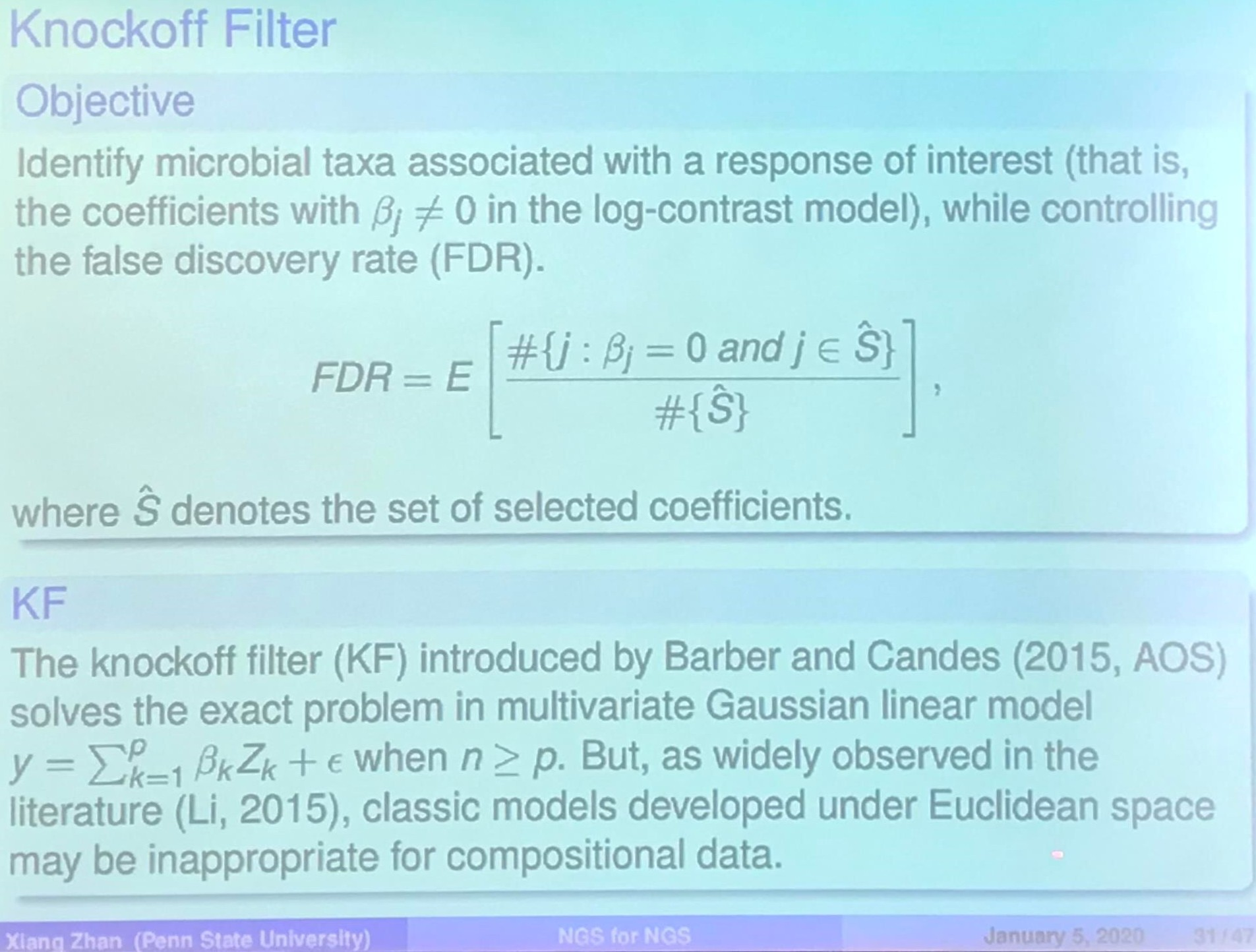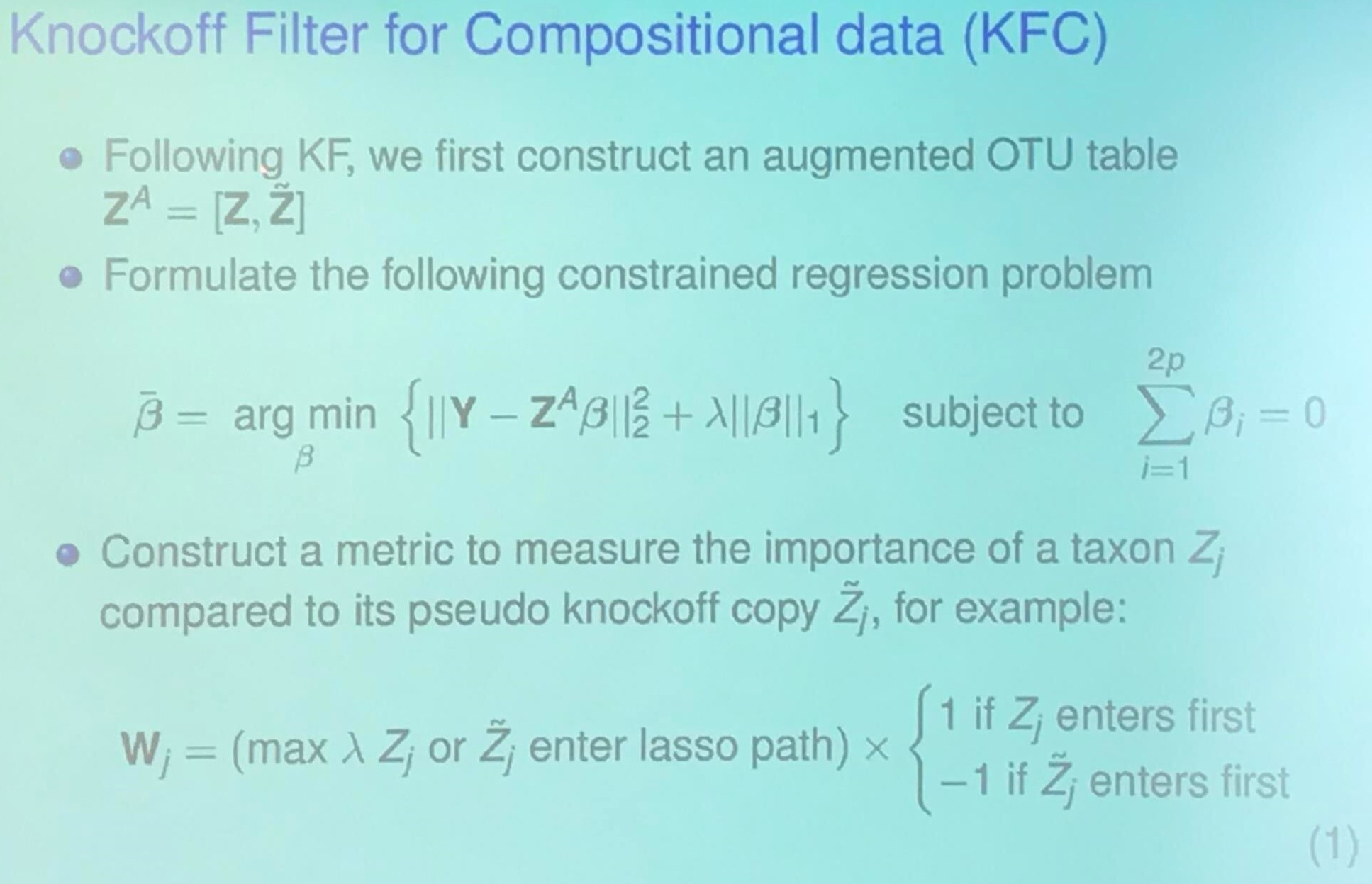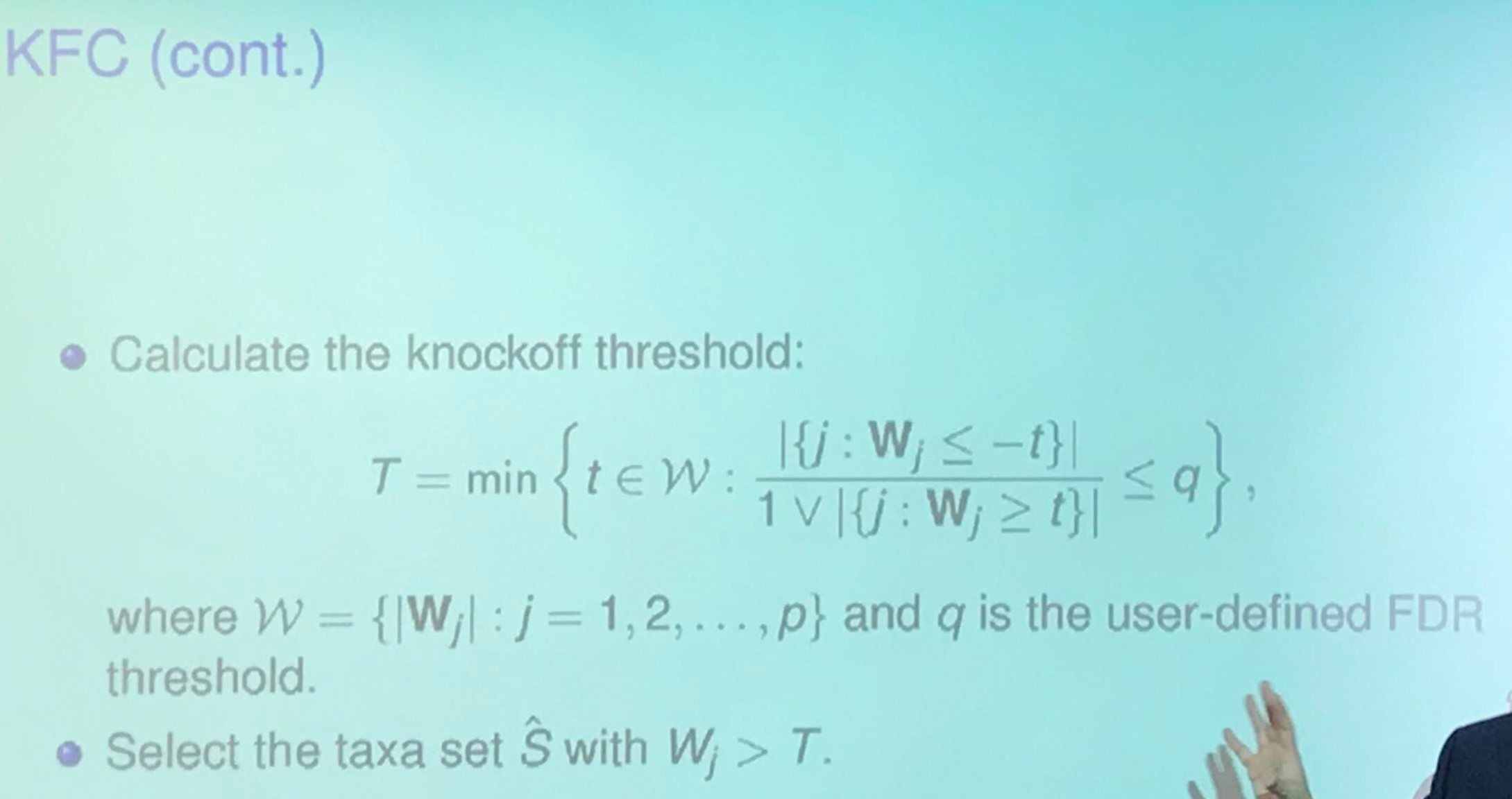Published in categories Seminar